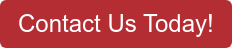# The Pump Toolbox Conversions

## Quick and easy on-the-go conversion formulas for centrifugal pumping applications.

Article written by FCX Performance, Inc

We know when it comes to pump maintenance, sometimes you must use conversions to get the answers you need for a proper pump assessment. Do you often find yourself searching the internet looking for formulas to fill in those blanks? The image below is our engineer’s “toolbox” of pump conversions. These conversions range from specific gravity to pressure and power. Using our toolbox, we’ve worked out a few of our most common conversions so that you can see how to work through them yourself. With a little plug and chug, you’ll be ordering parts and finding solutions to your pump issues faster than before.

## Section 1: How to Calculate Pressure Conversions

iimage

How to Convert PSI to TDH

Let’s say you need a pump to provide an additional pressure of 50 PSI to your system. However, your pump curve is only showing pressure as Total Dynamic Head or TDH. How do you convert PSI to TDH?

Following the pressure formula above, we have worked out the conversion of PSI to TDH using water as our fluid. Water’s specific gravity, or SG, is equal to 1.0.

First, take your PSI (50) and multiply it by the constant 2.31. Next, divide that number by your fluid’s specific gravity (1.0). Our answers come out to 115.5 TDH meaning, we need to find a pump curve that will provide us with 115.5 TDH at our required flow.

image

How to Convert TDH to PSI

We can also flip the pressure formula to calculate PSI. Using this equation can help us confirm what PSI pressure will be provided based on the pump’s curve which is typically given in TDH.

Based on the previous examples, we will use TDH equal to 115.5. If you divide our TDH by the constant 2.31, then multiply that number by our fluids SG (1.0), you’ll find that the pressure your pump will provide is 50 PSI.

## Section 2: How to Calculate Horsepower Conversion

image

How to calculate Hydraulic (Liquid) Horsepower

If you need to calculate the horsepower that your fluid is generating at a given flow and pressure, then you need to calculate your Hydraulic (Liquid) Horsepower. To do so, you will need to know your gallons per minute (GPM), TDH, and SG of your fluid.

In this example, our GPM is equal to 100, our TDH is equal to 115.5, and we will use the SG of water again which equals 1.0. First, multiply the GPM, TDH and SG. That total is then divided by the constant 3,960, which then makes the Hydraulic Horsepower equal to 2.92 HP.

image

How to calculate Brake Horsepower

If you’re looking to calculate the input power delivered by the motor to the pump, you need to calculate the Brake Horsepower. To do that you will need to know your GPM, TDH, SG, and your pump’s efficiency as a percentage (this can be found on your pump’s performance curve).

In this example, our GPM is equal to 100, our TDH is equal to 115.5, our SG is equal to 1.0, and we find that our pump’s efficiency is 85%. First, you will need to multiply GPM, TDH, and SG. That total is then divided by the product of our constant of 3,960 and your pump’s efficiency of 85%. This results in our Brake Horsepower equaling 3.43 BHP.

(Hint: the difference between BHP and LHP is the pump efficiency. You can also take the LHP and divide that by your pump’s efficiency to calculate BHP)

If you would like us to work through different problems, let us know what you want to see by reaching out to us!

### talk with an expert

Our engineers work to fulfil our customers’ pump needs each day. Even if it means working out the difficult problems so that your newly repaired pumps or customized pumping solution fit seamlessly into your system. Check your math with one of our experts today.